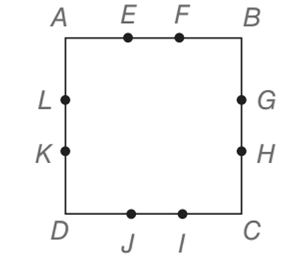Chapter 4.3, Problem 46EElementary Geometry For College St...

7th Edition
Alexander + 2 others
ISBN: 9781337614085

Solutions

Chapter
SectionElementary Geometry For College St...

7th Edition
Alexander + 2 others
ISBN: 9781337614085
Textbook Problem

The sides of square ABCD are trisected at the indicated points. If AB = 3, find the perimeter of(a) quadrilateral EGIK.(b) quadrilateral EHIL.To determine

(a)

To find:

The perimeter of quadrilateral EGIK.

Explanation

Calculation:

Given: ABCD is a square.

AB = 3

Now we have to find the perimeter of quadrilateral EGIK.

Both diagonals of EGIK are 3 each since they are the same lengths as sides of ABCD...

To determine

(b)

To find:

The perimeter of quadrilateral EHIL.

Still sussing out bartleby?

Check out a sample textbook solution.

See a sample solution

The Solution to Your Study Problems

Bartleby provides explanations to thousands of textbook problems written by our experts, many with advanced degrees!

Get Started

Find more solutions based on key concepts